# PSAT Math : How to subtract trinomials

## Example Questions

### Example Question #6 : Simplifying Polynomials

Evaluate the following: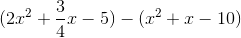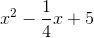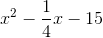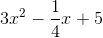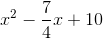Explanation:

To subtract these two trinomials, you first need to flip the sign on every term in the second trinomial, since it is being subtrated: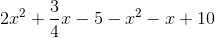Next you can combine like terms. You have two terms with, two terms with, and two terms with no variable:### Example Question #15 : Trinomials

Find the difference: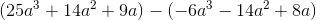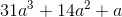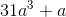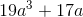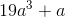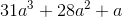Explanation:

Find the difference:Distribute the negative to the second trinomial: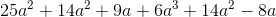Combine like terms:### Example Question #1 : How To Subtract Trinomials

Subtract: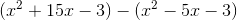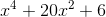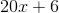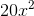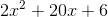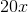When subtracting trinomials, first distribute the negative sign to the expression being subtracted, and then remove the parentheses: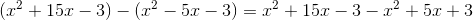Next, identify and group the like terms in order to combine them: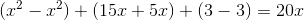.